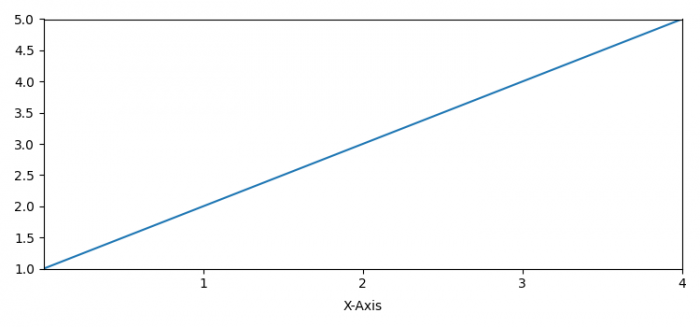# How to change the separation between tick labels and axis labels in Matplotlib?

To change the separation between tick labels and axis labels in Matplotlib, we can use labelpad in xlabel() method.

## Steps

• Set the figure size and adjust the padding between and around the subplots.
• Plot data points of a list using plot() method.
• Set the ticks on the axes.
• Set X and Y axes margins to 0.
• Set the X-axis label with labelpad.
• To display the figure, use show() method.

## Example

from matplotlib import pyplot as plt

plt.rcParams["figure.figsize"] = [7.50, 3.50]
plt.rcParams["figure.autolayout"] = True

plt.plot([1, 2, 3, 4, 5])

plt.xticks([1, 2, 3, 4, 5])
plt.margins(x=0, y=0)
plt.show()